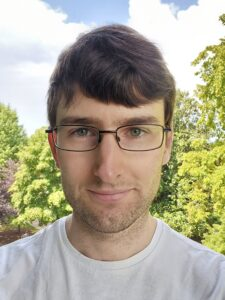# Samuel Shepherd, Ph.D. – September 2022 Postdoc Feature

Posted by on Wednesday, August 31, 2022 in Postdoc Features.

Written by Sam Shepherd, Ph.D.Symmetry occurs everywhere in the natural world, from the atomic structures of different materials, to the spherical shapes of stars and planets, to the bilateral symmetry present in many animals. I study group theory, which is the mathematical language that describes symmetry in all its myriad forms. After completing my PhD at the University of Oxford, I am now one year into my postdoc here at Vanderbilt, and I have had a great time so far. I am grateful for the support I have received from colleagues and mentors within the math department, as well as from the wider Vanderbilt community.

To get some insight into group theory and my research, let’s focus on a particular example. Take the standard square tiling of the plane (i.e. an infinite grid) and consider the symmetries of the plane that preserve this tiling. Any two symmetries can be combined to produce a third symmetry, e.g. if you translate one square to the left and then translate five squares to the right, the overall effect is translating four squares to the right. Or if you rotate 90° about a vertex and then translate one square to the right, the overall effect is rotating 90° about a different point (draw a diagram to figure out what point!). A group of symmetries is a collection of symmetries together with the information about how pairs of symmetries combine. The square tiling of the plane has many different groups of symmetries, e.g. the group of all symmetries, the group of translations, or the group of left-right translations.

A group of symmetries can be studied from two different viewpoints. On one hand you can study its geometry, e.g. you can classify the symmetries of the square tiling of the plane as translations, rotations, reflections and glide reflections. On the other hand you can study its algebra, where you forget what each symmetry looks like and you just consider how pairs of symmetries combine together – which can be recorded using a multiplication table. There is no direct correspondence between geometry and algebra, in fact groups of symmetries of different shapes can have the same algebra, e.g. the group of rotations of the cube has an identical multiplication table to the group of all symmetries of the tetrahedron!

In my research I study the interactions between geometry and algebra for a wide variety of different groups of symmetries. For instance, some shapes are rigid in the sense that all of their groups of symmetries have “similar algebra” (this can be made more precise but requires more technical language). One of my current projects centers around investigating this rigidity for a wide class of shapes. This project is joint with Alex Margolis (Vanderbilt University), Emily Stark (Wesleyan University) and Daniel Woodhouse (University of Oxford).

In my free time I like going cycling or hiking, and going to sports events.